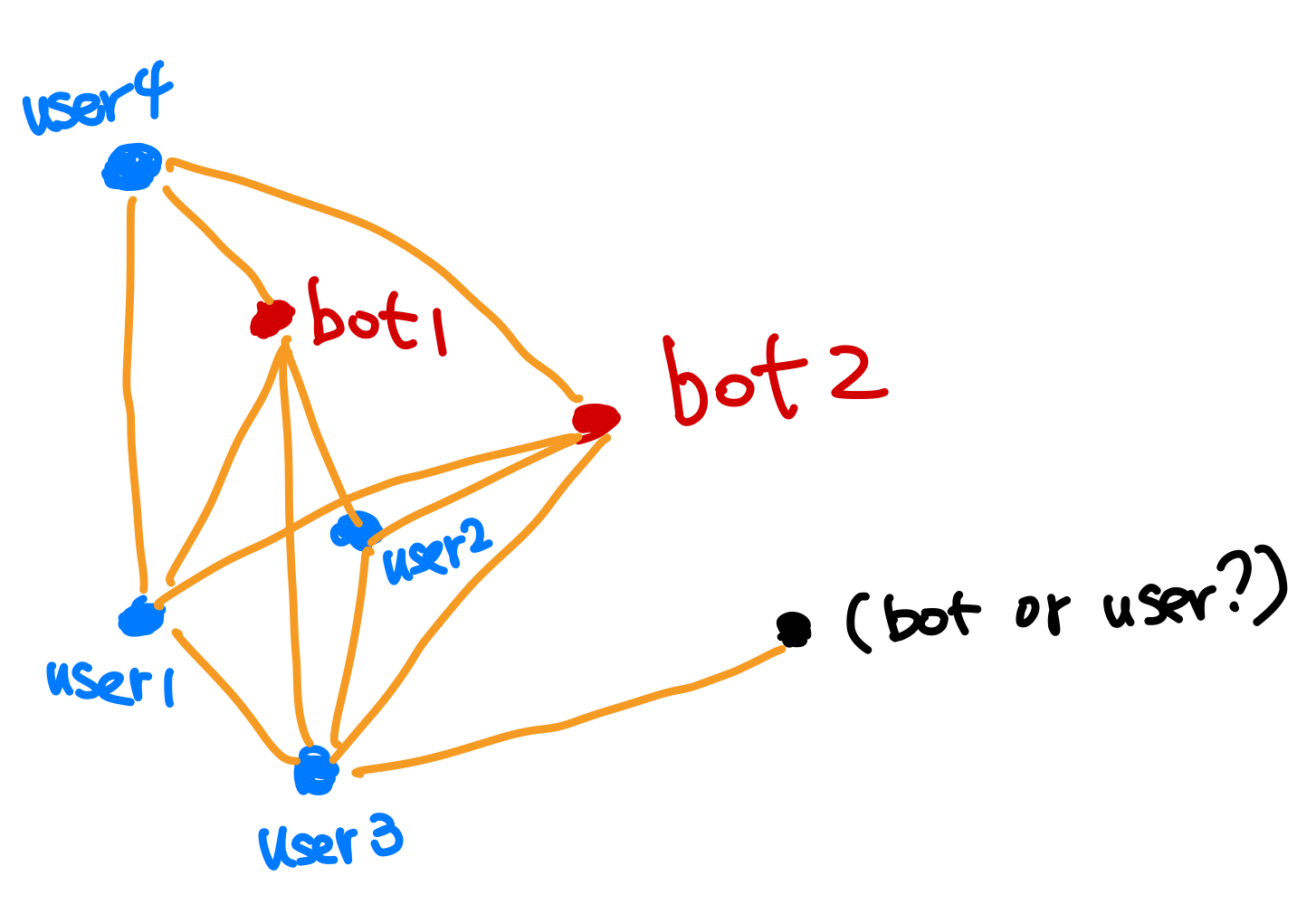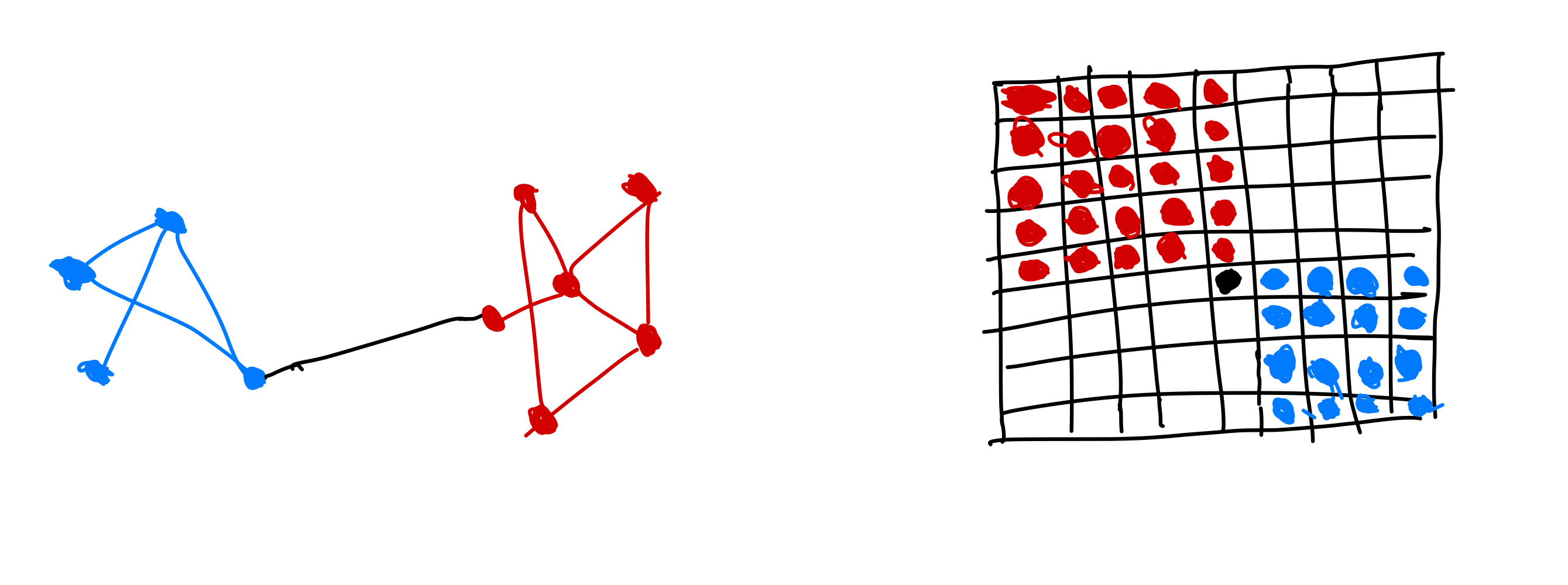Graphs can be used in many problem and there are many possible problems on graphs. We will mention a few popular problems on graphs12.

## Node ClassificationIs the user in black a bot or a normal user?Created based on the text in Hamilton2020

Given graph that has incomplete attribute labeling of the nodes, predict the attributes on the nodes.

The following concepts can be used to classify nodes.

Statement of the node classification problem

Given $\mathcal V_{\mathrm{train}}\subset \mathcal V$ on a graph, infer labels $y_u$ of node $u$, with $u\in \mathcal V\setminus \mathcal V_{\mathrm{train}}$.

## Relation Prediction

Also named as link prediction, graph completion.

Statement of the relation prediction problem

Given the nodes $\mathcal V$ on a graph $\mathcal G$, and $\mathcal E_{\text{train}}\subset \mathcal E$, predict other edges $\mathcal E \setminus \mathcal E_{\text{train}}$.

## Clustering and Community DetectionCommunities of collaboration networkEach node is an author, the edges indicate collaborations between the authors.

Assign nodes to groups. See for examples.

## Classification, Regression, and Clustering on Graph Level

Given some graphs, perform classification, regression, and clustering on the set of graphs, e.g., predict the toxicity of molecules.

Planted: by ;

L Ma (2021). 'Solving Problems on Graph', Datumorphism, 09 April. Available at: https://datumorphism.leima.is/wiki/graph/basics/ml-problems-on-graph/.